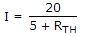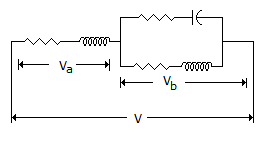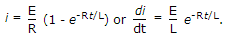# Electronics and Communication Engineering - Networks Analysis and Synthesis

1.

The three sides of an admittance triangle denote

 A. G, B, Y B. R, X, Y C. G, B, Z D. (R, Y, Z) or (R, X, Z)

Explanation:

2.

A step voltage E is applied to an R-L series circuit. At t = 0, the current in the circuit is

 A. E/R B. E/L C. infinity D. zero

Explanation:

Inductance does not allow the current to increase instantaneously.

3.

To find current in a resistance connected in a network, Thevenin's theorem is used VTH = 20 V and RTH = 5 Ω. The current through the resistance

 A. is 4 A B. is 4 A or less C. is less than 4 A D. may be 4 A or less or more than 4 A

Explanation:4.

In figure, the magnitude of VA. must be more than that of Va B. must be more than that of Vb C. must be more than that of Va or Vb D. can be equal to or less than or more than that of Va or Vb

Explanation:

V depends on magnitudes and phase angles of Va and Vb.

5.

A step voltage E is applied to a series R-L circuit. The rate of change of current is maximum at t =

 A. zero B. infinity C. L/R D. R/L